解决CocosCreator2.4.x的ABundle不能使用npm包的问题前言

Creator | Asset Bundle 全解析

@一下引擎组

问题重要吗?

EasyGameFramework的出发点就是要让编码更加Easy，更加爽。

复现问题

flag已经立了，说明我已经解决了。哈哈哈哈哈哈~ 它不可能倒下的~

github.com/AILHC/EasyG…

我简单讲一下我的测试逻辑

1. 加载abtest这个包：test/display-ctrl/abtest，然后将这个包里的ABTestModule注入模块管理器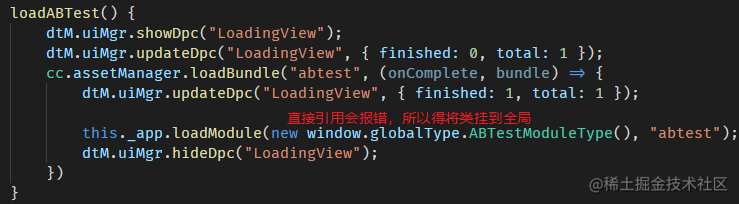2. 通过abtest模块的逻辑，显示abtest里的界面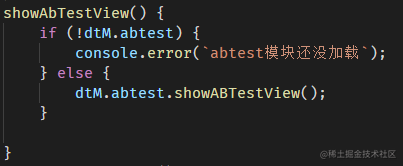3. ABTestModule注入全局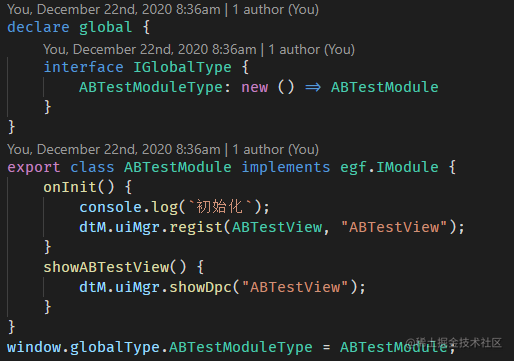4. ABTestView继承@ailhc/dpctrl-ccc包的NodeCtrl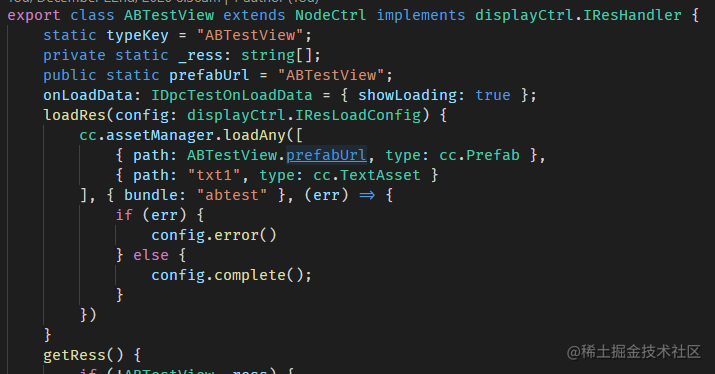万能的断点调试

1. 我们会发现，在分包的index.js找不到，只有两个包内的脚本模块对象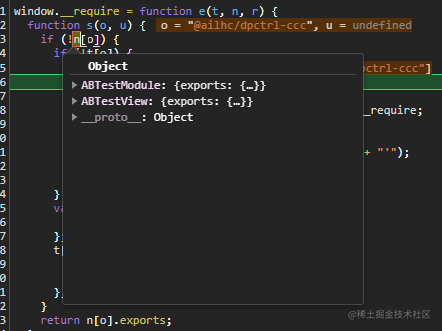2. 下一步。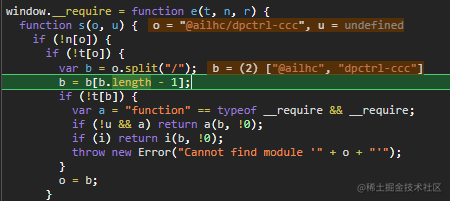· npm包名是没有./或者../的，大多是只有一个名字 比如xstate
· 有一些特殊的包名比如:@babel/core，比如@ailhc/egf-core

1. 继续跟踪，它进了一个require函数，然后跳转过去，发现这个index.js是main bundle里的

调试总结

• 主包main里的index.js有npm包模块，也可以引用
• 分包里的index.js如果引用了包外的脚本模块，则会向上main包查找，查不到就报错
• 而这个分包向主包查找模块的逻辑里，没考虑到npm包名的情况
• 主包和分包里的index.js的开头那部分都很像，像是模板

如何来解决这个问题呢？

首先得找到模板index.js开头

(function e(t, n, r) {
function s(o, u) {
if (!n[o]) {
if (!t[o]) {
var b = o.split('/');
b = b[b.length - 1];
if (!t[b]) {
var a = "function" == typeof __require && __require;
if (!u && a) return a(b, !0);
if (i) return i(b, !0);
throw new Error("Cannot find module '" + o + "'");
}
o = b;
}
var f = n[o] = {
exports: {}
};
t[o].call(f.exports, function (e) {
var n = t[o][e];
return s(n || e);
}, f, f.exports, e, t, n, r);
}
return n[o].exports;
}
var i = "function" == typeof __require && __require;
for (var o = 0; o < r.length; o++) s(r[o]);
return s;
})

然后呢，解决那个切掉我npm包名的逻辑

var b = o;
if (o.includes("./")) {
//内部代码
b = o.split("/");
b = b[b.length - 1];
} else {
//npm包代码
}

最后呢，因为主包中，npm包包名和模块对象的对应关系被转换了

(function e(t, n, r) {
function s(o, u, npmPkgName) {
if (!n[o]) {
if (!t[o]) {
var b = o;
if (o.includes("./")) {
//内部代码
b = o.split("/");
b = b[b.length - 1];
} else {
//npm包代码
}
if (!t[b]) {
var a = "function" == typeof __require && __require;
if (!u && a) return a(b, !0);
if (i) return i(b, !0);
throw new Error("Cannot find module '" + o + "'");
}
o = b;
}
var f = n[o] = {
exports: {}
};
t[o].call(f.exports, function (e) {
var n = t[o][e];
//判断是不是npm包，是就传包名
return s(n || e, undefined, !e.includes("./") ? e : undefined);
}, f, f.exports, e, t, n, r);
}
//判断是不是npm包，是就用包名作为key存一下模块引用
if (npmPkgName && n[o] && !n[npmPkgName]) {
n[npmPkgName] = n[o];
}
return n[o].exports;
}
var i = "function" == typeof __require && __require;
for (var o = 0; o < r.length; o++) s(r[o]);
return s;
})

最后

QQ 群: 1103157878

github: github.com/AILHC